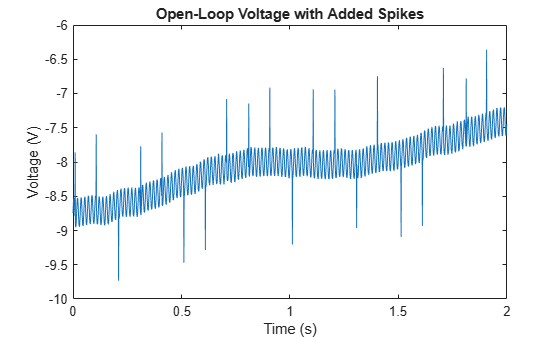## Remove Spikes from a Signal

Sometimes data exhibit unwanted transients, or spikes. Median filtering is a natural way to eliminate them.

Consider the open-loop voltage across the input of an analog instrument in the presence of 60 Hz power-line noise. The sample rate is 1 kHz.

```load openloop60hertz fs = 1000; t = (0:numel(openLoopVoltage) - 1)/fs;```

Corrupt the signal by adding transients with random signs at random points. Reset the random number generator for reproducibility.

```rng default spikeSignal = zeros(size(openLoopVoltage)); spks = 10:100:1990; spikeSignal(spks+round(2*randn(size(spks)))) = sign(randn(size(spks))); noisyLoopVoltage = openLoopVoltage + spikeSignal; plot(t,noisyLoopVoltage) xlabel('Time (s)') ylabel('Voltage (V)') title('Open-Loop Voltage with Added Spikes')````yax = ylim;`

The function `medfilt1` replaces every point of a signal by the median of that point and a specified number of neighboring points. Accordingly, median filtering discards points that differ considerably from their surroundings. Filter the signal, using sets of three neighboring points to compute the medians. Note how the spikes vanish.

```medfiltLoopVoltage = medfilt1(noisyLoopVoltage,3); plot(t,medfiltLoopVoltage) xlabel('Time (s)') ylabel('Voltage (V)') title('Open-Loop Voltage After Median Filtering') ylim(yax) grid```